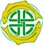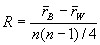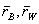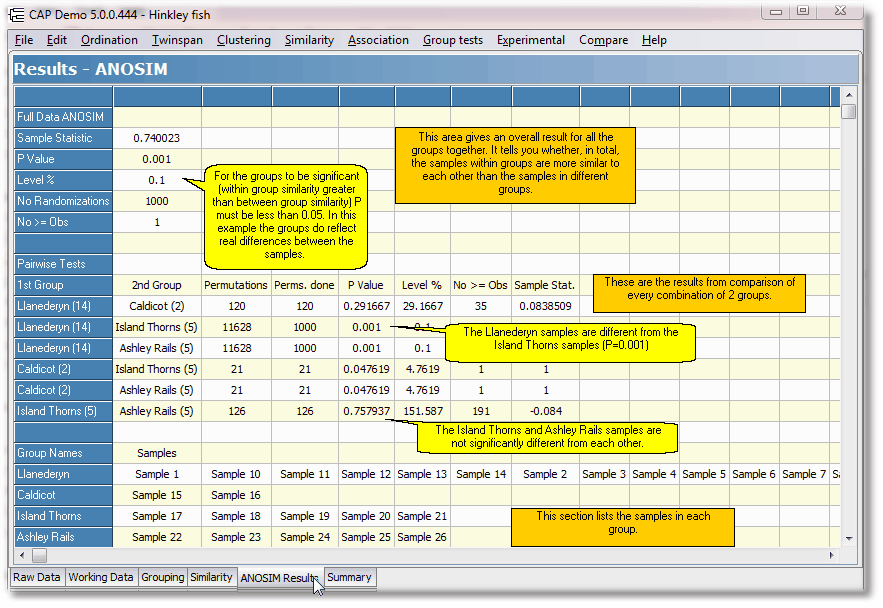﻿ Analysis of Similarity (ANOSIM)Analysis of Similarity (ANOSIM)
 To undertake this test you must first have defined the group membership of the individual samples (see Allocating samples to Groups).   This test was developed by Clark (1988, 1993) as a test of the significance of the groups that had been defined a priori. The idea is simple; if the assigned groups are meaningful, samples within groups should be more similar in composition than samples from different groups. The method uses the Bray-Curtis measure of similarity. The null hypothesis is therefore that there are no differences between the members of the various groups.   Clark (1988, 1993) proposed the following statistic to measure the differences between the groups:whereare the mean of the ranked similarity BETWEEN groups and WITHIN groups respectively and n is the total number of samples (objects).   R scales from +1 to -1. +1 indicates that all the most similar samples are within the same groups. R = 0 occurs if the high and low similarities are perfectly mixed and bear no relationship to the group. A value of -1 indicates that the most similar samples are all outside of the groups. While negative values might seem to be a most unlikely eventuality it has been found to occur with surprising frequency.   To test for significance, the ranked similarity within and between groups is compared with the similarity that would be generated by random chance. Essentially the samples are randomly assigned to groups 1000 times and R calculated for each permutation. The observed value of R is then compared against the random distribution to determine if it is significantly different from that which could occur at random.   If the value of R is significant, you can conclude that there is evidence that the samples within groups are more similar than would be expected by random chance.   The layout of the ANOSIM results is explained below: